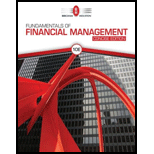# WACC Empire Electric Company (EEC) uses only debt and common equity. It can borrow unlimited amounts at an interest rate of r d = 9% as long as it finances at its target capital structure, which calls for 35% debt and 65% common equity. Its last dividend (D 0 ) was $2.20, its expected constant growth rate is 6%, and its common stock sells for$26. EEC’s tax rate is 25%. Two projects are available: Project A has a rate of return of 125% and Project B’s return is 11.5%. These two protects are equally risky and about as risky as the firm’s existing assets. a. What is its cost of common equity? b. What is the WACC? c. Which projects should Empire accept?### Fundamentals Of Financial Manageme...

10th Edition
Eugene F. Brigham + 1 other
Publisher: Cengage Learning
ISBN: 9781337902571

#### Solutions

Chapter
Section### Fundamentals Of Financial Manageme...

10th Edition
Eugene F. Brigham + 1 other
Publisher: Cengage Learning
ISBN: 9781337902571
Chapter 10, Problem 12P
Textbook Problem
3 views

## WACC Empire Electric Company (EEC) uses only debt and common equity. It can borrow unlimited amounts at an interest rate of rd = 9% as long as it finances at its target capital structure, which calls for 35% debt and 65% common equity. Its last dividend (D0) was $2.20, its expected constant growth rate is 6%, and its common stock sells for$26. EEC’s tax rate is 25%. Two projects are available: Project A has a rate of return of 125% and Project B’s return is 11.5%. These two protects are equally risky and about as risky as the firm’s existing assets. a. What is its cost of common equity? b. What is the WACC? c. Which projects should Empire accept?

a.

Summary Introduction

To identify: The cost of common equity.

Cost of Equity:

It is the cost of the company while raising finance by issuing equity. It is the earnings from the investment to the firm’s equity investors. It is the return to the stockholder holders’ equity investments.

### Explanation of Solution

Given,

Last dividend is $2.20 per share. Growth rate is 6% or 0.06. Current price of stock is$26.

Formula to calculate cost of common equity is,

r=D0(1+g)P0+g

Where,

• D0 is the last dividend.
• P0 is the current price of the stock.
• g is the constant growth rate.

Substitute $2.20 for D0,$26 for P0 and 0

b.

Summary Introduction

To determine: Weighted average cost of capital.

Weighted Average Cost of Capital (WACC):

It is the weighted average cost of all the sources through which a firm finances its capital. It is the rate that a company will pay to all for raising finance. It can be termed as the firm’s cost of capital. The company raises money through various sources such as common stock and preference share debt. The WACC is calculated by taking the relative weight of each item of capital structure.

c.

Summary Introduction

To identify: The project that should be accepted by E Company.

### Still sussing out bartleby?

Check out a sample textbook solution.

See a sample solution

#### The Solution to Your Study Problems

Bartleby provides explanations to thousands of textbook problems written by our experts, many with advanced degrees!

Get Started

Find more solutions based on key concepts
What is a logical DFD?

Accounting Information Systems

What were Elton Mayos conclusions from the Hawthorne Studies?

Foundations of Business (MindTap Course List)

Show that if A1, A2, and A3 are independent events, then P(A1 | A2 A3) 5 P(A1).

Probability and Statistics for Engineering and the Sciences

In what ways is economics a science?

Principles of Microeconomics (MindTap Course List)

What is inflation and what causes it?

Essentials of Economics (MindTap Course List)

BUILDING CREDIT COST INTO PRICES Your firm sells for cash only, but it is thinking of offering credit, allowing...

Fundamentals of Financial Management, Concise Edition (with Thomson ONE - Business School Edition, 1 term (6 months) Printed Access Card) (MindTap Course List)

Departmental operating income is the difference between a departments gross profit and its operating expenses.

College Accounting, Chapters 1-27 (New in Accounting from Heintz and Parry)